# Kerala Syllabus 8th Standard Maths Solutions Chapter 6 Construction of Quadrilaterals

## Kerala State Syllabus 8th Standard Maths Solutions Chapter 6 Construction of Quadrilaterals

Textbook Page No. 108

Construction Of Quadrilaterals Class 8 State Syllabus Question 1.
Can you draw these patterns of squares in your notebook?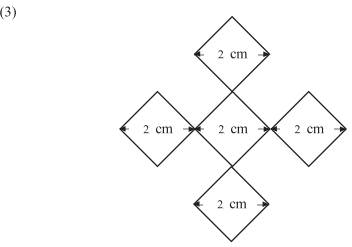Solution:
1. Draw a square of side 6 cm. Draw lines horizontally and vertically 2cm apart. Rub off unwanted parts. We get the required pattern.2. Draw a square of side 7 cm. In it draw horizontal and vertical lines at intervals of 4 cm, 2 cm and 1 cm. Erase the unwanted part. We get the required pattern.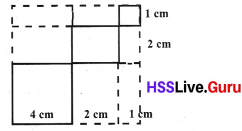3. Draw a square of diameter 6 cm. (Draw a circle of diameter 6 cm and draw two perpendicular diameters. Join their ends.) Mark the points on diagonal 2 cm a part. Join the points as in the following figure. Rub off the unwanted part. We get the required pattern.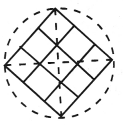Text Book Page No. 111

Class 8 Mathematics Construction Of Quadrilaterals Kerala Scert Solutions Question 2.
Draw the figures below in your notebook.Solution:
1. Draw a rectangle of diagonal 9 cm and angle between the diagonal and one side is 30°. Mark a point on the diagonal at a distance 6 cm from one end of the diagonal. Draw lines perpendicular to the sides of the rectangle through this point. Erase unwanted parts, we get the required pattern.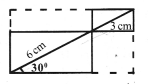2. Construct an equilateral triangle with side 3 cm.The other two sides of a triangle are made by equal diagonals of the rectangle. Then the diagonal of the rectangle is 6 cm. Draw a rectangle in a horizontal position with diagonals 6 cm and the angle between the diagonals 60°. Draw another rectangle of the same measure in the vertical position at the middle of the first rectangle. Draw the wanted part in bold lines. Erase unwanted part.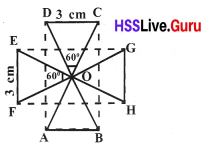Construction Of Quadrilaterals Class 8 Kerala Syllabus Question 3.
Draw a rectangleCBD, which di agonal (AB) is 6 cm and AC making an angle 30° with AB. The arc with centre at B and BC as radius and the arc with centre at A and AC as radi us meet at E. Complete ∆ AEB. Draw an arc with centre A and EB as radi us. Also draw arc with B as centre and AE as radius meet at F. Complete the rectangle AEBF.Text Book Page No. 114

8th Class Maths Construction Of Quadrilaterals Kerala Syllabus Question 3.
Draw a rhombus of diagonals 5.5 cm and 3 cm in your notebook.
Solution:
Draw a line of length 5.5 cm, and find its midpoint by drawing the perpendicular bisector. Mark the points on the upper and lower part of the bisector.
Mark the points on the upper and lower part of the bisector line at a distance 1.5 cm from the intersecting point of the first line and perpendicular bisector. Join these points to the end of the first line.Construction Of Quadrilaterals 8th Class Kerala Syllabus Question 4.
Draw also a rhombus of diagonals 5.5 cm and 3.5 cm.
Solution.
It is difficult to measure 1.75 cm (half of 3.5 cm) using scale, so draw a rectangle of 5.5 cm and breadth of 3.5 cm. By drawing the perpendicular bisectors find the midpoints of the sides. By joining the midpoints of the sides, we get a rhombus.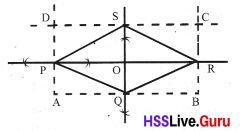Textbook Page No. 117

Class 8 Construction Of Quadrilaterals Kerala Syllabus Question 5.
Draw these figures
Solution:
1. Two equal rhombuses :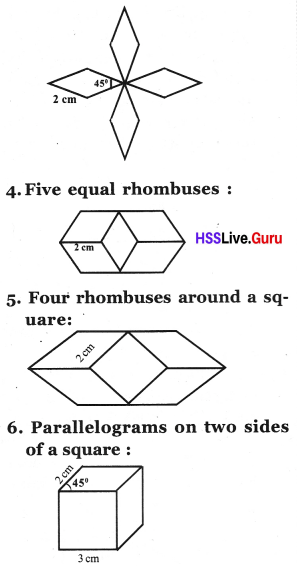Solution:
1. From the figure two sides of A BCD are equal, angles opposite these sides are also equal. We can calculate them as 50° each. In the same way find other angles in the figure.Draw a line BD vertically, 3 cm long. At D draw angles of 50° on both sides. At B also draw angles of 50° on both sides. Then we get a rhombus ABCD. Extend BC to G such that BC = CG and extend DC to E such that DC = CE. Draw GE. Draw angle of 50° at G and E to find F.

2. Draw a circle of radius 2 cm. Divide the centre of the circle into angles of 60° each. These lines meet the circle at the points A, B, C, D, E and F.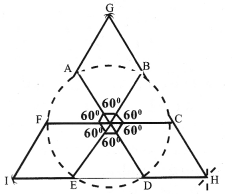Draw arcs of 2 cm from A and B to get G. Similarly find H and I. Draw the required parts and rub off unwanted parts.

Construction of Quadrilaterals Class 8 Question 3. Draw a circle of radius 2 cm. Mark the points A, B, C, D, E, F, G, and H on the circle by making 45° angles at the centre. Draw arc of 2 cm A and B to get I. Similarly find J, K and L. We get the required figure.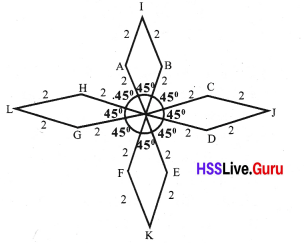4. Draw AC, 4 cm long and mark its midpoint B. Since all are rhombuses, ABI is a equilateral triangle. Its angle are 60° each.In the rhombus BCDJ, ∠ JBC = ∠JDC = 60°, ∠BCD = ∠BJD = 120°
In the rhombus BJFI, ∠IBJ =∠IFJ = 60°, ∠BJF = ∠FIB = 120°. Draw each rhombus and complete the pattern.

5. Draw a line AB, 4 cm long and mark its midpoint C. CJFI is a square, all its angle are 90° each. Also calculate ∠ICA = 45° and ∠CAH = 135°. Taking measures of the sides and angles draw each rhombus.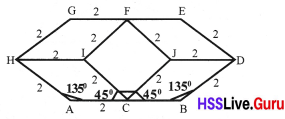6. Draw a square of side 3 cm. And draw two parallelograms with sides 3 cm, 2 cm and angle between them 45°, on the sides of the square.Text Book Page No. 124

Construction Of Quadrilaterals Class 8 Solutions Kerala Syllabus  Question 6.
Draw the figures below :
1. Three equal isosceles trapeziums: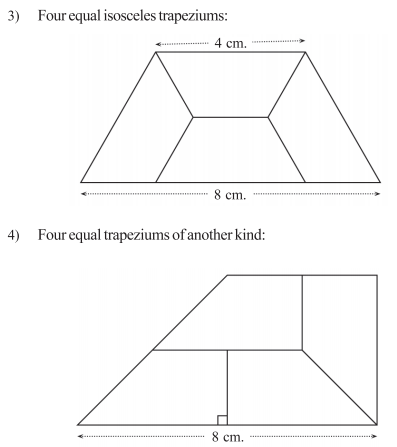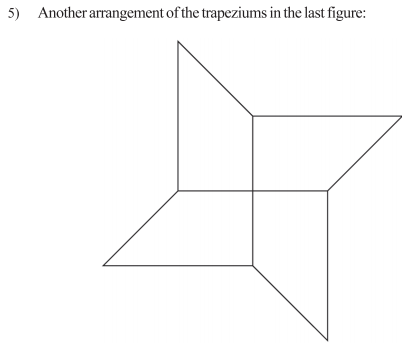Solution:
1. Draw DE, 5 cm long and mark G on DE such that DG = 2 cm (length of AB) ∆ ABC and ∆ DEF are equilateral triangles. Their each angle is 60°. Find B by drawing ∠EGB = ∠BEG = 30°. Draw ∠ADG = 30°and BA = 2 cm to get A. Now we got one trapezium. Draw the other two trapezium in the same way.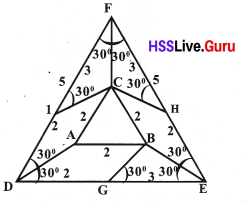2. Draw two circle of radius 1 cm and 3 cm with the same centre. Divide the circumferance of the both circle into 6 equal parts. And join them to obtain six equal isosceles trapeziums.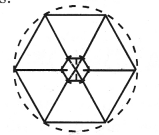3. Draw two parallel sides AB = 8 cm, CD = 4 cm. AE= FB = 2 cm, EF = 4 cm. Also GD = HC = 2 cm. ∠B + ∠B CD = 180°. The angles at C are equal. They are equal to B. So ∠B = $$\frac{180}{3}$$ = 60°. ∠A = 60°. Now draw the pattern.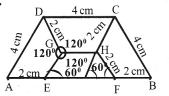4. Draw a rectangle with length 8 cm and breadth 4 cm. Divide this rectangle into two squares with side 4 cm. Consider one square and half of second. Mark the midpoint of the sides. Join as in the figure.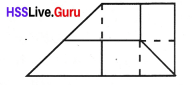5. Draw a square of side 8 cm. And draw lines horizontally and vertically 2cm apart. Complete the figure.Text Book Page No. 128

8th Class Maths Notes Kerala Syllabus Question 7.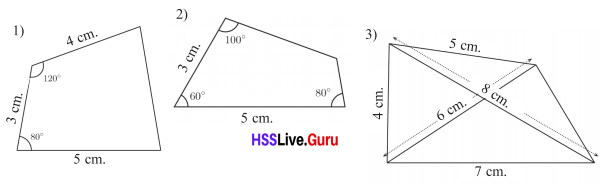Solution:
1.Draw AB = 5 cm. Draw AD such that ∠A = 80° and AD =3 cm. Mark C such that ∠D = 120° and DC = 4 cm. Join BC.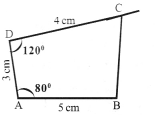2. Draw AB = 5 cm. Draw AD such that ∠A = 60° and AD = 3 cm. Mark point C such that ∠B = 80° and ∠D = 100°. Join DC and BC and complete the quadrilateral ABCD.3. Draw a ABD with AB = 7 cm, BD = 8 cm and AD = 4 cm. Mark the point C at a distance 6 cm from A and 5 cm from D. Join BC and CD.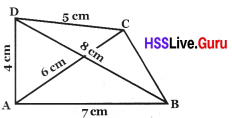Class 8 Maths Construction Of Quadrilaterals Kerala Syllabus Question 1.
In the figure, ABCD is a square whose diagonals intersect at O. If AD = 10 cm, find the length of BD and CD ?Solution:
Tn a square diagonal are equal and perpendicular bisectors of each other.
OD = 5 cm and OC = 5 cm.
CD2 = OD2 + OC2 = 52 +52 = 50 cm
CD = $$\sqrt{50}$$ = BD = $$5\sqrt{2}$$ cm.

Kerala Syllabus 8th Standard Maths Notes Question 2.
Draw quadrilateral PQRS, PQ = 7 cm, QR = 5 cm, RS= 4cm, ∠Q = 60° and ∠R = 140°.
Solution:
Draw PQ = 7 cm and draw QR of length 5 cm which makes an angle 60° with PQ. Draw RS such that RS = 4 cm and ∠R = 140°.8th Standard Maths Notes State Syllabus Question 3.
(a) Write any two peculiarities of the diagonals of a square.
(b) The length of a diagonal of a square is 7 cm. Draw the square.
Solution:
(a) The diagonals of a square are equal.
The diagonals bisect each other perpendicularly.
(b)Question 4.
In rhombus PQRS , PR = 7 cm and Question = 5 cm. Construct rhombus PQRS.
Solution:Question 5.
In the figure, ABCD is a parallelogram. ∠D = 80°. Find all other angles?Solution:
ABCD is a parallelogram
Opposite angles are equal ∠B = 80°.
Sum of the angles on the same side is 180°.
∠A + ∠B = 180°.
∠A = 180° – 80° = 100°
And ∠C = 100° (opposite angles are equal).

Question 6.
In the figure, ABCD is a parallelogram. Find x, y, z.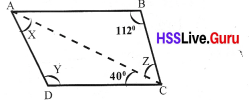Solution:
∠Y = 112° (opposite angles are equal)
In ADC, ∠x + ∠y + 40 = 180° (sum of angles in a triangle)
∠x + 112° + 40° = 180°
∠x = 180° – 152° = 28°
∠z = 28°(transversal alternate interior angles are equal).

Question 7.
Construct a quadrilateral ABCD, AB = 6 cm, BC = 3 cm, CD = 2 cm, AD = 4 cm and AC = 5 cm.
Solution:
Draw AB = 6 cm. Then find C by drawing arcs of radius 5 cm and 3 cm from A and B. Find D by drawing arcs of radius 4 cm and 2 cm from A and C. Join BC, CD and AD to get quadrilateral ABCD.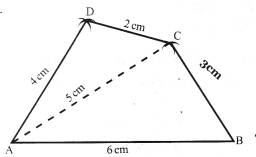Question 8.
Construct a quadrilateral ABCD, AB = 8 cm, BC = 6 cm, CD = 5.5 cm, DA = 3 cm and ∠B = 50°.
Solution:
Draw AB = 6 cm. Draw BC such that BC = 6 cm and ∠B = 50°. Draw an arc of radius 5.5 cm with C as centre and another arc of radius 3 cm with A as centre. Mark the point of intersection as D. Join CD and AD.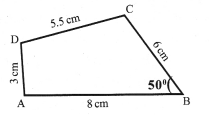Question 9.
In parallelogram ABCD, the diagonals AC and BD intersect at O. AC = 6.5 cm, BD = 7 cm and ∠AOB = 100°. Construct the parallelogram.
Solution:Question 10.
The diagonals of a rhombus are of lengths 16 cm and 12 cm. What is its perimeter?
Solution: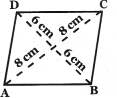In right angled AOB,
AO = 8 cm, BO = 6 cm, ∠AOB = 90°
AB2 = AO2 + BO2 = 82 + 62
= 64 + 36 = 100
Side, AB = $$\sqrt{100}$$ = 10 cm
Perimeter = 4 × 10 = 40 cm.

Question 11.
Draw the following patterns, a. 6 equal rhombuses :
(a) 6 equal rhombuses: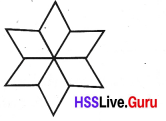(b) 3 equal rhombuses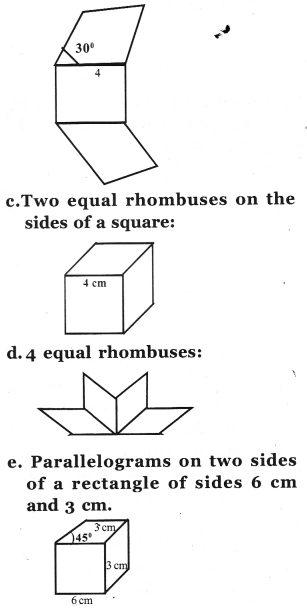Solution:
(a) Draw a circle of radius 2 cm with centre O. Divide the centre of circle into angles of 60° each. These lines meet the circle at the points A, B, C, D, E and F. Draw arc of 2 cm from A and B to get G. In the same way find H, I, J, K, L. Draw needed part.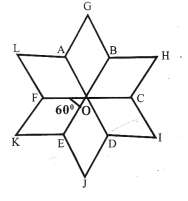(b) Draw square of side 4 cm. Draw rhombuses with side 4 cm and an gle 30° on top and bottom side of the square. Complete the figure.

(c) Angles around the point at which three rhombuses joined together is 120° each. Since one angle of the rhombus is 120°, another angle 60°. Draw three rhombuses with side 4 cm and angle 60°. Complete the figure.

(d) Draw a semicircle of radius 2 cm with centre O. Divide the centre of circle into angles of 45° each .These lines meet the circle at the point A, B, C, D and E. Draw arc of 2 cm from A and B to get F. In the same way find G, H and I. Complete the figure.(e) Draw a rectangle of length 6 cm and breadth 3 cm. Draw two rhombuses on both side of the rectangle which makes angle 45° and 135° with length and breadth respectively.

Question 12.
In a parallelogram ABCD, find x, y, z from the adjoining figure.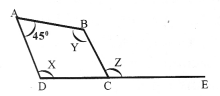Solution:
ABCD is a parallelogram,
∠C = 45° (Opposite angles are equal)
∠C + Z = 180° (linear pair)
Z = 180° – 45° = 135°
45° + Y = 180° (sum of the angles on the same side is 180°)
Y = 180° – 45° = 135°
sinceY = 135°
X = 135°(Opposite angles are equal)